Contemporary Mathematics

# 5.6Quadratic Equations with Two Variables with Applications

Contemporary Mathematics5.6 Quadratic Equations with Two Variables with Applications

Figure 5.41 The Gateway Arch in St. Louis, Missouri (credit: modification of work "Gateway Arch - St. Louis - Missouri" by Sam valadi/Flickr, CC BY 2.0)

### Learning Objectives

After completing this section, you should be able to:

1. Multiply binomials.
2. Factor trinomials.
3. Solve quadratic equations by graphing.
4. Solve quadratic equations by factoring.
5. Solve quadratic equations using square root method.
7. Solve real world applications modeled by quadratic equations.

In this section, we will discuss quadratic equations. There are several real-world scenarios that can be represented by the graph of a quadratic equation. Think of the Gateway Arch in St. Louis, Missouri. Both ends of the arch are 630 feet apart and the arch is 630 feet tall. You can plot these points on a coordinate system and create a parabola to graph the quadratic equation.

### Identify Polynomials, Monomials, Binomials and Trinomials

You have learned that a term is a constant, or the product of a constant and one or more variables. When it is of the form $axmaxm$, where $aa$ is a constant and $xmxm$ is a positive whole number, it is called a monomial. Some examples of monomial are 8, $-2x2-2x2$, $4y34y3$, and $11z11z$.

A monomial or two or more monomials combined by addition or subtraction is a polynomial. Some examples include: $b+11b+11$, $4y2−7y+24y2−7y+2$, and $4x4+x3+8x2−9x+14x4+x3+8x2−9x+1$. Some polynomials have special names, based on the number of terms. A monomial is a polynomial with exactly one term (examples: 14, $8y28y2$, $-9x3y5-9x3y5$, and $−13−13$). A binomial has exactly two terms (examples: $a+ 7a+ 7$, $4b−54b−5$, $y2−16y2−16$, and $3x3−9x23x3−9x2$), and a trinomial has exactly three terms (examples: $x2−7x+12x2−7x+12$, $9y2+2y−89y2+2y−8$, $6m4−m3+8m6m4−m3+8m$, and $x4+3x2−1x4+3x2−1$).

Notice that every monomial, binomial, and trinomial is also a polynomial. They are just special members of the “family” of polynomials and so they have special names. We use the words monomial, binomial, and trinomial when referring to these special polynomials and just call all the rest polynomials.

### Multiply Binomials

Recall multiplying algebraic expressions from Algebraic Expressions. In this section, we will continue that work and multiply binomials as well. We can use an area model to do multiplication.

### Example 5.47

#### Multiply Binomials

Multiply $(x+2)(x+3)(x+2)(x+3)$.

1.
Multiply (x + 3)\,(x + 1).

### Example 5.48

#### Multiplying More Complex Binomials

Multiply $(2x+7)(3x−5)(2x+7)(3x−5)$.

1.
Multiply (x - 3)\,(2x + 1).

### Who Knew?

#### They Are Teaching Multiplication of Binomials in Elementary School

Manipulatives are often used in elementary school for students to experience a hands-on way to experience the mathematics they are learning. Base Ten Blocks, or Dienes Blocks, are often used to introduce place value and the operation of whole numbers. When multiplying two-digit numbers, students can make an array to visualize the Distributive Property. Figure 5.44 shows the value of each Base Ten Block and Figure 5.45 shows how to multiply 17 and 23 using an area model and Base Ten Blocks. You can see how this helps students visualize the multiplication using the Distributive Property. Consider how $(10+7)(20+3)(10+7)(20+3)$ can easily extend to $(10+x)(20+x)(10+x)(20+x)$ in algebra!

Figure 5.44 The Value of Each Base Ten Block
Figure 5.45 How to Multiply 17 and 23 Using an Area Model and Base Ten Blocks

### Factoring Trinomials

We’ve just covered how to multiply binomials. Now you will need to “undo” this multiplication—to start with the product and end up with the factors. Let us review an example of multiplying binomials to refresh your memory.

$(x+2)(x+3)=x2+5x+6(x+2)(x+3)=x2+5x+6$

To factor the trinomial means to start with the product, $x2+5x+6x2+5x+6$, and end with the factors, $(x+2)(x+3)(x+2)(x+3)$. You need to think about where each of the terms in the trinomial came from. The first term came from multiplying the first term in each binomial. So, to get $x2x2$ in the product, each binomial must start with an $xx$.

$x2+5x+6(x )(x )x2+5x+6(x )(x )$

The last term in the trinomial came from multiplying the last term in each binomial. So, the last terms must multiply to 6. What two numbers multiply to 6? The factors of 6 could be 1 and 6, or 2 and 3. How do you know which pair to use? Consider the middle term. It came from adding the outer and inner terms. So the numbers that must have a product of 6 will need a sum of 5.

We’ll test both possibilities and summarize the results in the following table, which will be very helpful when you work with numbers that can be factored in many different ways.

Factors of 6 Sum of Factors
1, 6
$1+6=71+6=7$
2, 3
$2+3=52+3=5$

We see that 2 and 3 are the numbers that multiply to 6 and add to 5. We have the factors of $x2+5x+6x2+5x+6$. They are $(x+2)(x+3)(x+2)(x+3)$.

$x2+5x+6product(x+2)(x+3)factorsx2+5x+6product(x+2)(x+3)factors$

You can check if the factors are correct by multiplying. Looking back, we started with $x2+5x+6 x2+5x+6$, which is of the form $x2+bx+cx2+bx+c$, where $b=5b=5$ and $c=6c=6$. We factored it into two binomials of the form $(x+m)(x+m)$ and $(x+n)(x+n)$.

$x2+5x+6x2+bx+c(x+2)(x+3)(x+m)(x+n)x2+5x+6x2+bx+c(x+2)(x+3)(x+m)(x+n)$

To get the correct factors, we found two number $mm$ and $nn$ whose product is $cc$ and sum is $bb$. With the area model (Figure 5.46), start with an empty box and then put in the $x2x2$ term and $cc$.

Figure 5.46

Continue by putting in two terms that add up to $5x:2x5x:2x$ and $3x 3x$ (Figure 5.47):

Figure 5.47

Then you find the terms of the binomials on the top and side (Figure 5.48):

Figure 5.48

### Example 5.49

#### Factoring Trinomials

Factor $x2+7x+12x2+7x+12$.

1.
Factor x^{2} + 6x + 8.

### Example 5.50

#### Factoring More Complex Trinomials

Factor $x2-11x+28x2-11x+28$.

1.
Factor: {x^2} - 16x + 63.

### Solving Quadratic Equations by Graphing

We have already solved and graphed linear equations in Graphing Linear Equations and Inequalities, equations of the form $Ax+By=CAx+By=C$. In linear equations, the variables have no exponents. Quadratic equations are equations in which the variable is squared. The following are some examples of quadratic equations:

$x2+5x+6=03y2+4y=1064u2−81=0n(n+1)=42x2+5x+6=03y2+4y=1064u2−81=0n(n+1)=42$

The last equation does not appear to have the variable squared, but when we simplify the expression on the left, we will get $n2+nn2+n$. The general form of a quadratic equation is $ax2+bx+c=0ax2+bx+c=0$, where $a,b,a,b,$ and $cc$ are real numbers, with $a≠0a≠0$. Remember that a solution of an equation is a value of a variable that makes a true statement when substituted into the equation. The solutions of quadratic equations are the values of the variables that make the quadratic equation $ax2+bx+c=0ax2+bx+c=0$ true.

To solve quadratic equations, we need methods different than the ones we used in solving linear equations. We will start by solving a quadratic equation from its graph. Just like we started graphing linear equations by plotting points, we will do the same for quadratic equations. Let us look first at graphing the quadratic equation $y=x2y=x2$. We will choose integer values of $xx$ between $−2−2$ and 2 and find their $yy$ values, as shown in the table below.

$y=x2y=x2$
$xx$ $yy$
0 0
1 1
$−1−1$ 1
2 4
$−2−2$ 4

Notice when we let $x=1 x=1$ and $x=−1x=−1$, we got the same value for $yy$.

$y=x2y=12y=1y=x2y=(−1)2y=1y=x2y=12y=1y=x2y=(−1)2y=1$

The same thing happened when we let $x=2x=2$ and $x=−2x=−2$. Now, we will plot the points to show the graph of $y=x2y=x2$. See Figure 5.49.

Figure 5.49

The graph is not a line. This figure is called a parabola. Every quadratic equation has a graph that looks like this. When $y=0y=0$ the solution to the quadratic $y=x2y=x2$ is 0 because $x2=0x2=0$ at $x=0x=0$.

### Example 5.51

Graph $y=x2-1 y=x2-1$ and list the solutions to the quadratic equation.

1.
Graph y = - \,{x^2}.

### Example 5.52

#### Solving a Quadratic Equation From Its Graph

Find the solutions of $y=x2+5x+4y=x2+5x+4$ from its graph (Figure 5.51).

Figure 5.51

1.
Find the solutions of y = {x^2} + x - 6 from its graph.
Figure 5.52

### Solving Quadratic Equations by Factoring

Another way of solving quadratic equations is by factoring. We will use the Zero Product Property that says that if the product of two quantities is zero, it must be that at least one of the quantities is zero. The only way to get a product equal to zero is to multiply by zero itself.

### Example 5.53

#### Solving a Quadratic Equation by Factoring

Solve $(x+1)(x-4)=0(x+1)(x-4)=0$.

1.
Solve (x - 2)(x + 5) = 0.

### Example 5.54

#### Solve Another Quadratic Equation by Factoring

Solve $x2+2x−8=0x2+2x−8=0$.

1.
Solve {x^2} + 2x-15 = 0.

### Checkpoint

Be careful to write the quadratic equation in standard form first. The equation must be set equal to zero in order for you to use the Zero Product Property! Often students start in Step 2 resulting in an incorrect solution. For example, $x2+2x−15=-7 x2+2x−15=-7$ cannot be factored to $(x−3)(x+5)=−7 (x−3)(x+5)=−7$ and then solved by setting each factor equal to $−7−7$.

The correct way to solve this quadratic equation is to set it equal to zero FIRST: $x2+2x−15+7=−7+7x2+2x−15+7=−7+7$ which becomes $x2+2x−8=0 x2+2x−8=0$, then continue to factor. See the table below for the correct way to apply the Zero Product Property.

 $x2+2x−15=−7x2+2x−15=−7$ $x2+2x−15=−7x2+2x−15=−7$ Step 1 Skipped $x2+2x−15+7=−7+7x2+2x−8=0x2+2x−15+7=−7+7x2+2x−8=0$ Step 2 $(x−3)(x+5)=−7(x−3)(x+5)=−7$ $(x−2)(x+4)=0(x−2)(x+4)=0$ Step 3 $x−3=−7x−3=−7$ $x+5=−7x+5=−7$ $x−2=0x−2=0$ $x+4=0x+4=0$ Step 4 $x=−4x=−4$ $x=−12x=−12$ $x=2x=2$ $x=−4x=−4$ Step 5 $(−4)2+2(−4)−15=16−8−15=−7(−4)2+2(−4)−15=16−8−15=−7$ $(−12)2+2(−12)−15=144−24−1=105≠−7(−12)2+2(−12)−15=144−24−1=105≠−7$ $(2)2+2(2)−8=4+4−8=0(2)2+2(2)−8=4+4−8=0$ $(−4)2+2(−4)−18=16−8−8=0(−4)2+2(−4)−18=16−8−8=0$

### Solving Quadratic Equations Using the Square Root Property

We just solved some quadratic equations by factoring. Let us use factoring to solve the quadratic equation $x2=9x2=9$.

 Step 1: Put the equation in standard form. $x2−9=0x2−9=0$ Step 2: Factor the left side. $(x+3)(x-3)=0(x+3)(x-3)=0$ Step 3: Use the Zero Product Property. $x+3 =0x+3 =0$ $x−3=0x−3=0$ Step 4: Solve each equation. $x=−3x=−3$ $x=3x=3$ Step 5: Combine the two solutions into $±±$ $x=±3x=±3$

The solution is read as “$xx$ is equal to positive or negative 3.”

What happens when we have an equation like $x2=7x2=7$? Since 7 is not a perfect square, we cannot solve the equation by factoring. These equations are all of the form $x2=kx2=k$. We define the square root of a number in this way: If $n2=mn2=m$, then $nn$ is a square root of $mm$. This leads to the Square Root Property.

### Example 5.55

#### Using the Square Root Property to Solve a Quadratic Equation

Solve using the square Root Property: $x2=169x2=169$.

1.
Solve using the Square Root Property: {x^2} = 25.

### Example 5.56

#### Using the Square Root Property to Solve Another Quadratic Equation

Solve using the Square Root Property: $144q2=25144q2=25$.

1.
Solve using the Square Root Property: 25{p^2} = 49.

This last method we will look at for solving quadratic equations is the quadratic formula. This method works for all quadratic equations, even the quadratic equations we could not factor! To use the quadratic formula, we substitute the values of $aa$, $bb$, and $cc$ into the expression on the right side of the formula. Then, we do all the math to simplify the expression. The result gives the solution(s) to the quadratic equation.

### Example 5.57

Solve using the quadratic formula: $x2-6x+5=0x2-6x+5=0$.

1.
Solve using the quadratic formula: {a^2} - 2a-15 = 0.

### Example 5.58

Solve using the quadratic formula: $2x2+9x−5=02x2+9x−5=0$.

1.
Solve using the quadratic formula: 3{y^2} - 5y + 2 = 0.

### Solving Real-World Applications Modeled by Quadratic Equations

There are problem solving strategies that will work well for applications that translate to quadratic equations. Here’s a problem-solving strategy to solve word problems:

Step 1: Read the problem. Make sure all the words and ideas are understood.

Step 2: Identify what we are looking for.

Step 3: Name what we are looking for. Choose a variable to represent that quantity.

Step 4: Translate into an equation. It may be helpful to restate the problem in one sentence with all the important information. Then, translate the English sentence into an algebra equation.

Step 5: Solve the equation using good algebra techniques.

Step 6: Check the answer in the problem and make sure it makes sense.

Step 7: Answer the question with a complete sentence.

### Example 5.59

#### Finding Consecutive Integers

The product of two consecutive integers is 132. Find the integers.

1.
The product of two consecutive odd integers is 240. Find the integers.

Were you surprised by the pair of negative integers that is one of the solutions? In some applications, negative solutions will result from the algebra, but will not be realistic for the situation.

### Example 5.60

#### Finding Length and Width of a Garden

A rectangular garden has an area 15 square feet. The length of the garden is 2 feet more than the width. Find the length and width of the garden.

1.
A rectangular sign has an area of 30 square feet. The length of the sign is 1 foot more than the width. Find the length and width of the sign.

### WORK IT OUT

#### Completing the Square

Recall the two methods used to solve quadratic equations of the form $ax2+bx+c:ax2+bx+c:$ by factoring and by using the quadratic formula. There are, however, many different methods for solving quadratic equations that were developed throughout history. Egyptian, Mesopotamian, Chinese, Indian, and Greek mathematicians all solved various types of quadratic equations, as did Arab mathematicians of the ninth through the twelfth centuries. It is one of these Arab mathematicians' methods that we wish to investigate with this activity.

Muhammad ibn Musa al-Khwarizmi was employed as a scholar at the House of Wisdom in Baghdad, located in present day Iraq. One of the many accomplishments of Al-Khwarizmi was his book on the topic of algebra. In that book, he asks, “What must be the square which, when increased by ten of its own roots, amounts to 39?” Al-Khwarizmi, like many Arab mathematicians of his time, was well versed in Euclid's Elements. Like Euclid, he viewed algebra very geometrically, and thus had a geometric approach to solving a problem like the one above. In his approach, he used a method which today we refer to as completing the square.

His description of the solution method for the above problem is: halve the number of roots, which in the present instance yields 5. This you multiply by itself; the product is 25. Add this to 39; the sum is 64. Now take the root of this which is 8, and subtract from it half the number of the roots, which is 5; the remainder is 3. This is the root of the square which you sought for. Thus the square is 9.

 So, what does all of this mean? Al-Khwarizmi would start with a square of unknown length of side (we will label the side length $xx$). See Figure 5.55 So, this square has area $x2x2$Figure 5.55 $xx$ He would then proceed to halve the number of roots (i.e., there are 10 roots by which the square is increasing) to get 5; this he would add to the first square. See Figure 5.56 The area of the two new pieces added into the original square are both $5x5x$. At this point, we have $x2+10x=39x2+10x=39$.Figure 5.56 Now Al-Khwarizmi needed to “complete the square” by adding into the drawing a small square. See Figure 5.57 This square has an area of 25.Figure 5.57

$x2+10x+25=39+25x2+10x+25=39+25$, or $x2+10x+25=64x2+10x+25=64$.

Notice that the completed square has side length $x+5x+5$, so the large square has area $(x+5)2(x+5)2$. (Notice algebraically that the left half of the equation $x2+10x+25=64x2+10x+25=64$ factors to $(x+5)2=64.)(x+5)2=64.)$ This means the area of large square equals 64. If $(x+5)2=64(x+5)2=64$, then $x+5=8x+5=8$; so $xx$ must be equal to 3 or $−13−13$ to make this true. Note that Al-Kwarimi would not have considered the possibility of a negative solution, since he approached the solution geometrically, and negative distances do not exist.

48.
Which quadratic equation equals \left( {x-3} \right)\left( {x + 5} \right)?
1. {x^2}-15
2. {x^2}-3x + 15
3. {x^2}-2x-15
4. {x^2} + 2x-15
49.
Which product is equal to {x^2}-8x + 15?
1. \left( {x-3} \right)\left( {x + 5} \right)
2. \left( {x-3} \right)\left( {x-5} \right)
3. \left( {x + 3} \right)\left( {x + 5} \right)
4. \left( {x + 3} \right)\left( {x-5} \right)
50.
The graph shown is the graph of which quadratic equation?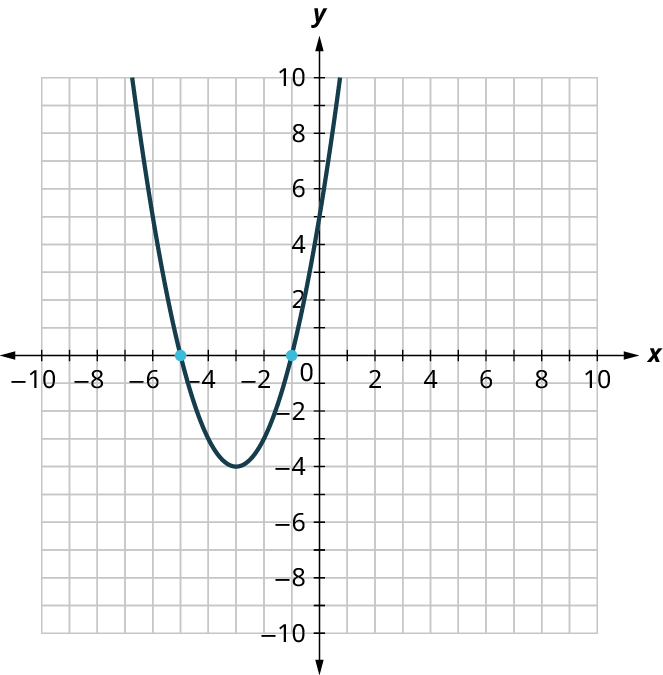1. {x^2}-x + 5 = 0
2. {x^2}-5x + 1 = 0
3. {x^2} + 6x + 5 = 0
4. {x^2}-4x + 5 = 0
51.
What is the solution to {x^2}-49 = 0?
1. x = 7
2. {x = -7}
3. {x = \pm 49}
4. {x = \pm 7}
52.
{x^2}-5x + 5 = 0 can be factored to \left( {x-1} \right)\left( {x-5} \right).
1. True
2. False
53.
{x^2}-5x + 5 = 0 can be solved using the square root method.
1. True
2. False
54.
{x^2}-5x + 5 = 0 can be solved using the quadratic formula.
1. True
2. False
55.
{x^2}-5x + 5 = 0 can be graphed as: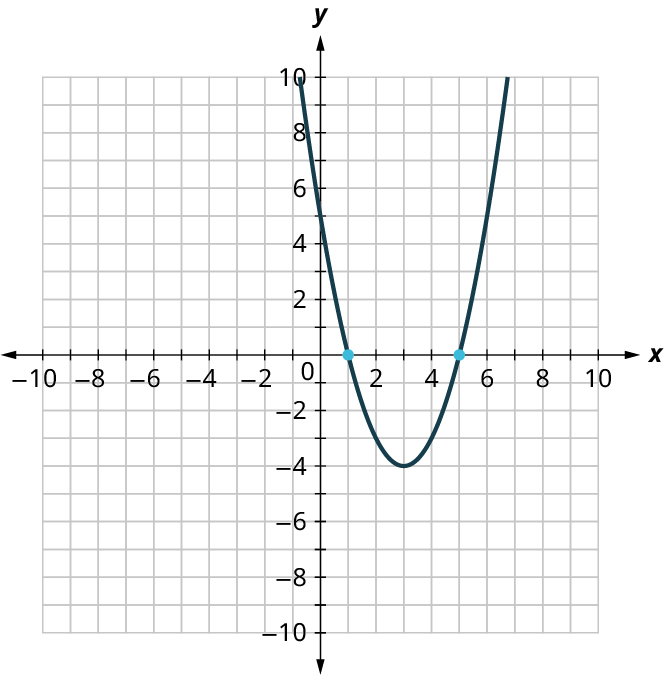1. True
2. False
56.
Using the square root method, find the solutions to {x^2}-5x + 5 = 0.

### Section 5.6 Exercises

For the following exercises, multiply the binomials.
1.
(w + 5)(w + 7)
2.
(y + 9)(y + 3)
3.
(p + 11)(p - 4)
4.
(q + 4)(q - 8)
5.
(x + 8)(x + 3)
6.
(2x - 1)(x + 6)
For the following exercises, factor the trinomials.
7.
{a^2} - 5a - 14
8.
{n^2} + 10n + 24
9.
{u^2} - 16
10.
{x^2} + 4x - 21
11.
{y^2} - 8y + 15
12.
{x^2} - 25
For the following exercises, solve the quadratic equations by graphing.
13.
Graph and list the solutions to the quadratic equation {x^2} - 5x - 14=0.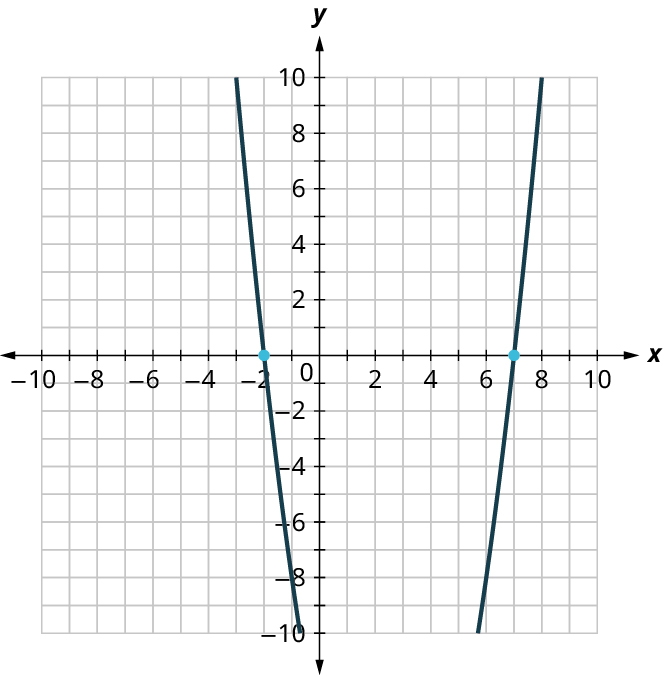14.
Graph and list the solutions to the quadratic equation {x^2} + 10x + 24=0.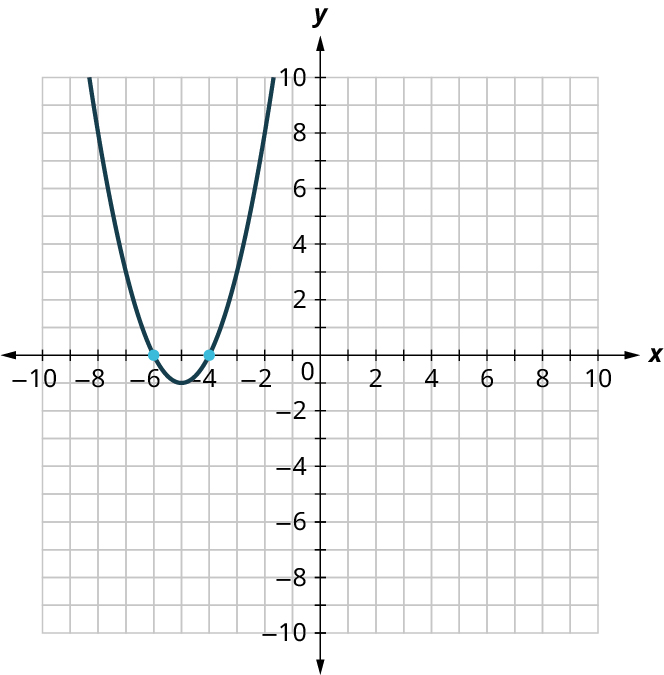15.
Graph and list the solutions to the quadratic equation {x^2} - 25=0.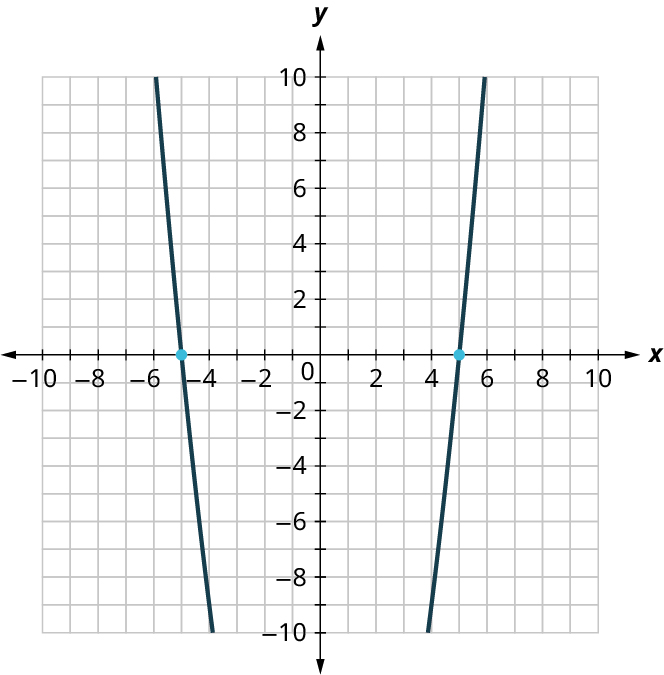16.
Find the solutions of y = {x^2} + 2x - 15 from its graph.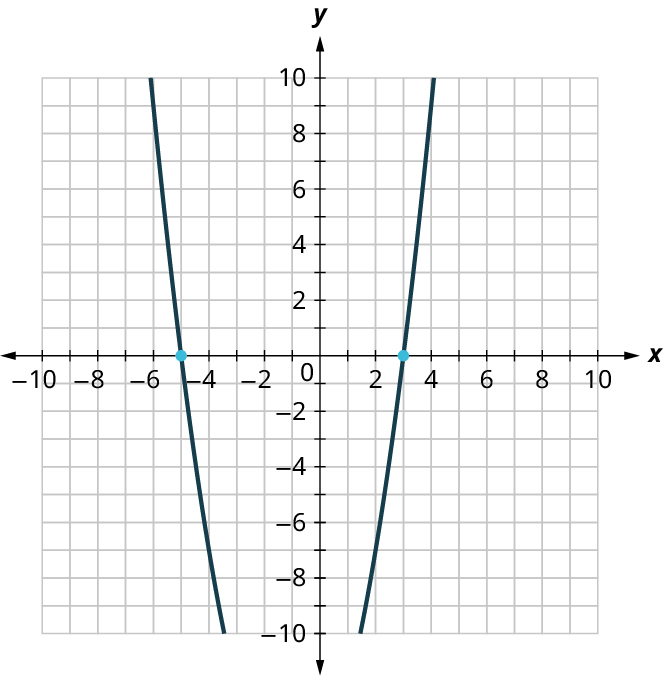17.
Find the solutions of y = {x^2} + 6x + 8 from its graph.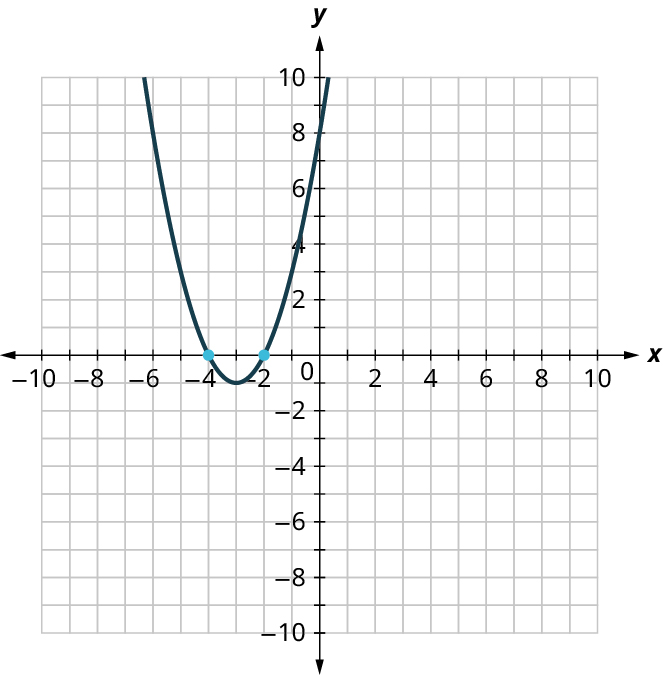18.
Find the solutions of y = {x^2} - 9x + 14 from its graph.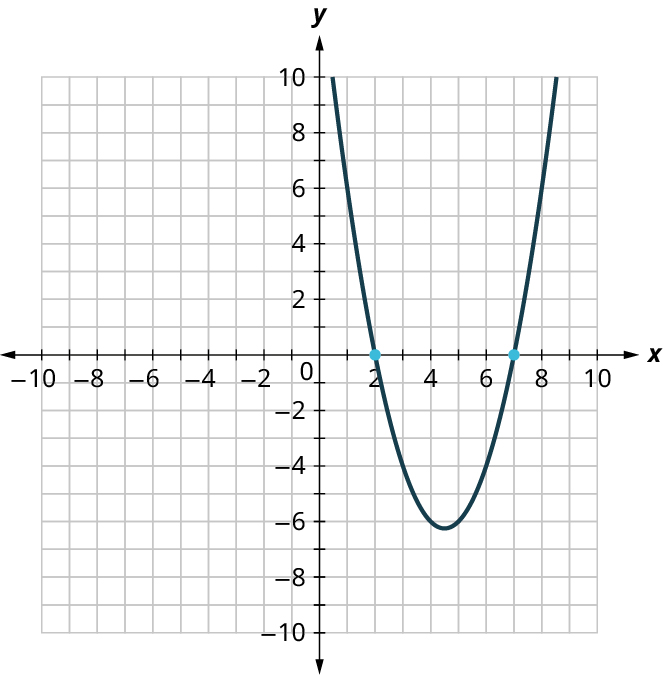For the following exercises, solve the quadratic equation by factoring.
19.
{x^2} + 7x + 12 = 0
20.
{y^2} - 8y + 15 = 0
21.
{n^2} - 5n + 6 = 0
22.
{a^2} + 2a = a
23.
{b^2} = - b
24.
{x^2} - 9x + 14 = 0
For the following exercises, solve the quadratic equation using the square root method.
25.
{x^2} = 16
26.
49{m^2} = 144
27.
625 = {x^2}
For the following exercises, solve the quadratic equation using the quadratic formula.
28.
16{p^2} = 24p - 9
29.
{m^2} - 2m = 1
30.
4{x^2} - 2x - 3 = 0
31.
{y^2} - 6y + 7 = 0
32.
{x^2} - 3x - 7 = 0
33.
2{x^2} - 4x - 11 = 0
34.
The product of two consecutive odd integers is 99. Find the integers.
35.
The product of two consecutive even integers is 168. Find the integers.
36.
A rectangular patio has an area of 180 square feet. The width of the patio is three feet less than the length. Find the length and width of the patio.
For the following exercises, use the Pythagorean Theorem: In any right triangle, where a and b are the lengths of the legs and c is the length of the hypotenuse, as shown in the given figure, {a^2} + {b^2} = {c^2}.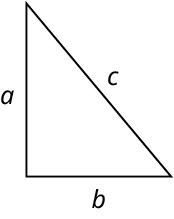37.
Justine wants to put a deck in the corner of her backyard in the shape of a right triangle, as shown in the given figure. The hypotenuse will be 17 feet long. The length of one side will be 7 feet less than the length of the other side. Find the lengths of the sides of the deck.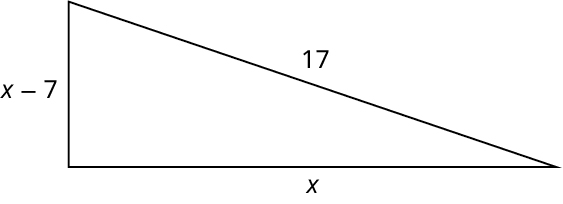38.
A boat’s sail is a right triangle. The length of one side of the sail is 7 feet more than the other side. The hypotenuse is 13. Find the lengths of the two sides of the sail.
39.
The sun casts a shadow from a flagpole. The height of the flagpole is three times the length of its shadow. The distance between the end of the shadow and the top of the flagpole is 21 feet. Find the length of the shadow and the length of the flagpole. Round to the nearest tenth of a foot.
40.
Rene is setting up a holiday light display. He wants to make a “tree” in the shape of two right triangles and has two 10-foot strings of lights to use for the sides. He will attach the lights to the top of a pole and to two stakes on the ground. He wants the height of the pole to be the same as the distance from the base of the pole to each stake. How tall should the pole be? Round to the nearest tenth of a foot.